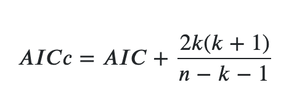# The equation of the AICc used in GWR

255
2
08-11-2022 11:29 AMby
New Contributor

Does anyone know the equation of the corrected AIC (AICc) used in the GWR implementation? Is it the simple version of the AICc as shown below?where n is the number of samples and k is the number of parameters.

In the implementation of the GWmodel R package, the following form is used:Thank you very much!

Tags (3)
1 Solution

Accepted SolutionsbyEsri Contributor

Hello,

We used the b version, the same as the GWmodel R package for AICc calculation in our GWR implementation.

Thanks.

2 RepliesbyEsri Contributor

Hello,

We used the b version, the same as the GWmodel R package for AICc calculation in our GWR implementation.

Thanks.by
New Contributor